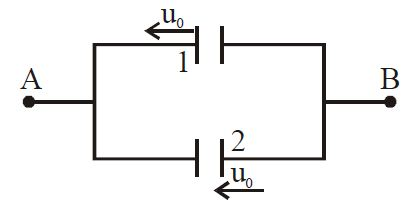# Moving Plates Capacitor!Two identical capacitors having plate separation $1 \text{ mm}$ are connected parallel to each other across points $A$ & $B$ as shown in figure. Total charge of $4 \mu C$ is imparted to the system by connecting a battery across $A$ & $B$ and battery is removed. Now first plate of first capacitor and second plate of second capacitor starts moving with constant velocity $u_0=3 \text{ m/s}$ towards left. Find the magnitude of current (in $\text{mA}$) flowing in the loop initially.

×